Announcements

# MOSFET (Si/SiC) Forum Discussions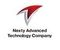Level 2Level 2

# How to correctly calculate the loss of MOSFETs used in PFC

Could you let me how to calculate the loss of MOSFET used in PFC?

For example, in the case of MOSFETs used in DC/DC, etc., the IDS and VDS of the MOSFET repeat regular switching.

When calculating losses from real waveforms, it is generally necessary to calculate turn-on loss, conduction loss, and turn-off loss.

For an accurate calculation, the total loss could be calculated by dividing the IDS and VDS into linear sections, integrating at each section, calculating the power loss per section, and adding them together.

On the other hand, I think the basic idea is the same for PFC, but in the case of PFC, the input current is controlled to be sinusoidal, so the IDS flowing in the MOSFET also changes every cycle.

In this case, how should the MOSFET loss generally be calculated?

Thanks.

1 SolutionModeratorModerator

# Re: How to correctly calculate the loss of MOSFETs used in PFC

Hello Fujioka-san,

Thank you for posting on Infineon Community.

Calculating the losses of a power MOSFET in a PFC is not simple.
There are several papers that propose different calculation methods.
However, based on your definition of switching losses, I understand that a simplistic approach (trigonometrical) should be enough in this case.
Such that the switching losses are assumed as a triangle: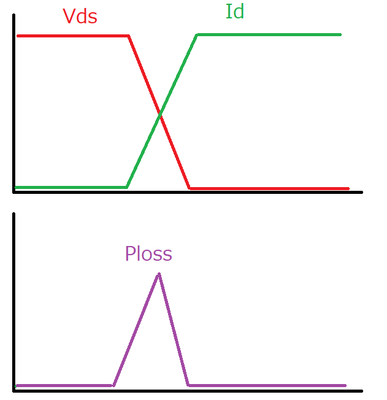Depending on the PFC type, the equations will vary.
The simplest calculation is for the critical conduction mode PFC, as it only has turn-off losses.
The output voltage is assumed to be constant and the ripple negligible.

Conduction losses:
As the current waveform is a triangle, Id is not constant.
Therefore, the following common expression needs to be integrated for a switching period.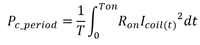After some substitution and replacing by sinusoidal expressions, we integrate for a half-period AC.
As a result, the conduction losses can be calculated by using the formula below.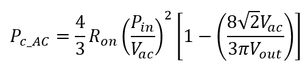Switching losses: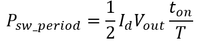Substituting with the definition of a switching period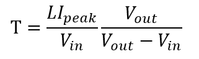Then, we need to integrate for a half-period AC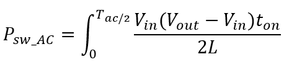With the following relations, we can substitute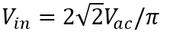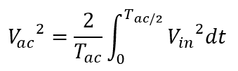And as a result, the switching losses can be calculated by using the formula below.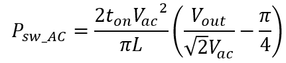In reality, the "ton" time will vary with the phase of the AC line, which means that we can expect some error from the equation due to this.

If accurate losses need to be calculated, I recommend creating a simulation of your specific PFC design.

Infineon provided SPICE models for all power devices, as well as PLECS models for certain products.

Alternatively, you can find a PFC simulation circuit on our online simulator:

TINA CLOUD

Best regards,

PabloModeratorModerator

# Re: How to correctly calculate the loss of MOSFETs used in PFC

Hello Fujioka-san,

Thank you for posting on Infineon Community.

Calculating the losses of a power MOSFET in a PFC is not simple.
There are several papers that propose different calculation methods.
However, based on your definition of switching losses, I understand that a simplistic approach (trigonometrical) should be enough in this case.
Such that the switching losses are assumed as a triangle:Depending on the PFC type, the equations will vary.
The simplest calculation is for the critical conduction mode PFC, as it only has turn-off losses.
The output voltage is assumed to be constant and the ripple negligible.

Conduction losses:
As the current waveform is a triangle, Id is not constant.
Therefore, the following common expression needs to be integrated for a switching period.After some substitution and replacing by sinusoidal expressions, we integrate for a half-period AC.
As a result, the conduction losses can be calculated by using the formula below.Switching losses:Substituting with the definition of a switching periodThen, we need to integrate for a half-period ACWith the following relations, we can substituteAnd as a result, the switching losses can be calculated by using the formula below.In reality, the "ton" time will vary with the phase of the AC line, which means that we can expect some error from the equation due to this.

If accurate losses need to be calculated, I recommend creating a simulation of your specific PFC design.

Infineon provided SPICE models for all power devices, as well as PLECS models for certain products.

Alternatively, you can find a PFC simulation circuit on our online simulator:

TINA CLOUD

Best regards,

Pablo